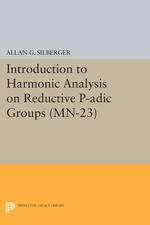# Introduction to Harmonic Analysis on Reductive P-adic Groups. (MN-23): Based on lectures by Harish-Chandra at The Institute for Advanced Study, 1971-73

Allan J. Silberger
Pages: 380
https://www.jstor.org/stable/j.ctt130hkdj

1. Front Matter
(pp. None)
2. FOREWORD
(pp. i-i)

These notes represent the writer's attempt to organize and comprehend the mathematics communicated to him by Harish-Chandra, both in public lectures and private conversations, during the years 1971-1973. They offer the reader an ab initio introduction to the theory of harmonic analysis on reductive p-adic groups. Besides laying the foundations for a theory of induced representations by presenting Jacquet's theory, the Bruhat theory, the theory of the constant term, and the Maass-Selberg relations, these notes develop the theory of the Schwartz space on a p-adic group and the theory of the Eisenstein integral in complete detail. They also give the...

3. ACKNOWLEDGMENTS.
(pp. i-i)
(pp. ii-iv)
5. Chapter 0. On the Structure of Reductive p-adic Groups.
(pp. 1-13)

The theory to be developed in the five later chapters of these notes depends upon the structure theory for reductive groups with points in a p-adic field. Fortunately, this theory has been worked out in detail (cf., [2a], [2c] for the essentially algebraic aspects and [4c] for the directly related topological part). In this chapter we very briefly review only those facts from the structure theory which we shall need later. The reader may consult the references both for proofs and more details.

If G is any group and H a subgroup, we write ZG(H)[NG(H)] for the centralizer [normalizer] of...

6. Chapter 1. Generalities Concerning Totally Disconnected Groups and Their Representations.
(pp. 14-77)

The intention of this chapter, violated in the two appendices to §1.2, is to present all the ideas needed in these notes which do not depend, either for their formulations or for their proofs, upon the theory of algebraic groups.

A t. d. space or totally disconnected space is a Hausdorff space which has a countable basis consisting of open compact sets. It follows that a t. d. space is locally compact. Subordinate to any open covering of a totally disconnected space, there is a covering of the space by a countable union of disjoint open compact sets. Any closed...

7. Chapter 2. Jacquet's Theory, Bruhat's Theory, the Elementary Theory of the Constant Term.
(pp. 78-115)

In this chapter and all remaining chapters of these notes we assume that G is the set of Ω-points of a connected reductive Ω-group G, Ω being a non-archimedean local field. For the properties of G which we shall need the reader should consult Chapter 0.

The symbol Z will always denote the split component of G, i.e., the set of Ω-points of the largest Ω-split torus Z lying in the center of G.

The chapter opens (§2.1) with a structure theorem for "infinitesimal" neighborhoods of the identity in G. In many applications this result replaces the differential operators and...

8. Chapter 3. Exponents and the Maass-Selberg Relations.
(pp. 116-146)

The purpose of this chapter is to prepare what we need and to prove the Maass-Selberg relations (Theorem 3. 5. 3) in the case where the inducing representation is supercuspidal. A more general version of this theorem (Theorem 4. 6. 3), in which the inducing representation is only required to be square integrable, is proved in the next chapter.

Let G be, as usual, the set of Ω-points of a reductive Ω-group and let Z be the split component of G. For U a complex vector space consider$\text{f}\in \cal{A}(\text{G}:\text{U})$. According to Corollary 1.10.2, f is Z-finite, so the subspace...

9. Chapter 4. The Schwartz Spaces.
(pp. 147-220)

The Schwartz spaces, 𝒞(G) and 𝒞*(G), will be introduced and studied in this chapter. We shall see that 𝒞(G)[𝒞*(G)] is a complete locally convex Hausdorff space. The space 𝒞(G) is an algebra under convolution on G; for every$\chi \in {\hat{\text{Z}}}$there is a space 𝒞*(G, χ) ⊂ 𝒞*(G) such that 𝒞*(G, χ) is an algebra under convolution on G/Z. There are inclusion mappings, continuous in the relevant topologies;$\text{C}_{\text{c}}^{\infty }(\text{G})\hookrightarrow {\cal{C}}(\text{G})\hookrightarrow {{\text{L}}^{2}}(\text{G})[\text{C}_{\text{c}}^{\infty }(\text{G},\chi )\hookrightarrow {\cal{C}}_{*}(\text{G},\chi )\hookrightarrow {{\text{L}}^{2}}(\text{G},\chi )]$. The K-finite matrix coefficients of the discrete series of G lie in 𝒞*(G), wave packets in 𝒞(G) (cf. §5. 5. 1). Indeed, it is a nontrivial problem to construct wave packets...

10. Chapter 5. The Eisenstein Integral and Applications.
(pp. 221-361)

We fix, for the whole chapter, a maximal split torus A0of G and A0-good maximal compact subgroup K of G.

Let A be an A0-standard torus, M = ZG(A), and ω ∈ ℰ2(M). If ω is unramified, then we have seen that the theory of induced representations provides us with a class$\text {C}_{\text {M}}^{\text {G}}(\omega )\in \cal {E}(\text {G})$. Let (V,τ) be a smooth unitary double rep representation of K. Then the map f ↦ fP= ΣfP, sfrom$\cal {A}(\text {C}(\omega),\tau)$to$\underset{\text{s}\in \text{W}(\text{A})}{\mathop{\oplus }}\,{\cal {A}}({{\omega }^{\text {s}}},{{\tau }_{\text {M}}})$gives rise to the Maass-Selberg relations. The Eisenstein integral, ψ ↦ E(P:ψ) (P ∈ ℙ(A)), maps$\cal{A}(\omega ,{{\tau }_{\text{M}}})$to$\cal {A}(\text {C}(\omega ),\tau )$....

11. References.
(pp. 362-364)
12. SELECTED TERMINOLOGY.
(pp. 365-369)
13. SELECTED NOTATIONS.
(pp. 370-371)
14. Back Matter
(pp. 372-372)